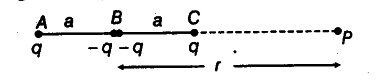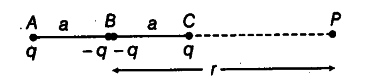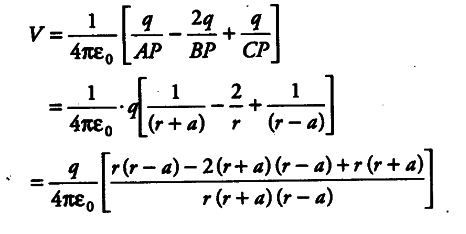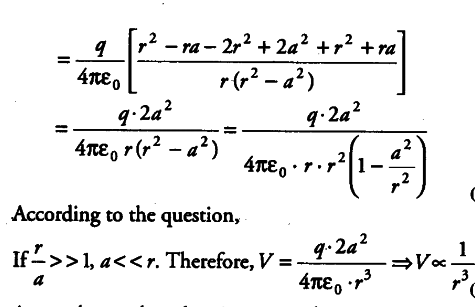# Given figure shows a charge array known as an electric quadrupole

Given figure shows a charge array known as an electric quadrupole. For a point on the axis of the quadrupole, obtain the dependence of potential on r for r/a >> 1 and contrast your results with that due to an electric dipole and an electric monopole (i.e. a single charge).Given, AC = 2a, BP = r
AP = r + a and PC = r - aThe potential at P is V.
.’. V = Potential at Pdue to A + Potential at due to B + Potential at P due to CIn case of electric monopole, V ∝ 1 / r.

Then, we conclude that for larger r, the electric potential due to a quadrupole is inversely proportional to the cube of the distance r, while due to an electric dipole, it is inversely proportional to the square of r and inversely proportional to the distance r for a monopole.